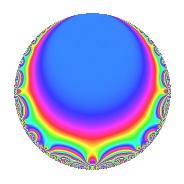# Properties

 Label 6027.2.a.lLevel 6027 Weight 2 Character orbit 6027.a Self dual Yes Analytic conductor 48.126 Analytic rank 0 Dimension 2 CM No Inner twists 1

# Related objects

## Newspace parameters

 Level: $$N$$ = $$6027 = 3 \cdot 7^{2} \cdot 41$$ Weight: $$k$$ = $$2$$ Character orbit: $$[\chi]$$ = 6027.a (trivial)

## Newform invariants

 Self dual: Yes Analytic conductor: $$48.1258372982$$ Analytic rank: $$0$$ Dimension: $$2$$ Coefficient field: $$\Q(\sqrt{3})$$ Coefficient ring: $$\Z[a_1, \ldots, a_{11}]$$ Coefficient ring index: $$1$$ Fricke sign: $$-1$$ Sato-Tate group: $\mathrm{SU}(2)$

## $q$-expansion

Coefficients of the $$q$$-expansion are expressed in terms of $$\beta = \sqrt{3}$$. We also show the integral $$q$$-expansion of the trace form.

 $$f(q)$$ $$=$$ $$q - q^{3} -2 q^{4} -2 \beta q^{5} + q^{9} +O(q^{10})$$ $$q - q^{3} -2 q^{4} -2 \beta q^{5} + q^{9} + ( 4 + \beta ) q^{11} + 2 q^{12} + ( 2 + 2 \beta ) q^{13} + 2 \beta q^{15} + 4 q^{16} + 5 q^{17} + ( 4 - 2 \beta ) q^{19} + 4 \beta q^{20} + ( 2 + 2 \beta ) q^{23} + 7 q^{25} - q^{27} -3 \beta q^{29} + ( 4 + \beta ) q^{31} + ( -4 - \beta ) q^{33} -2 q^{36} - q^{37} + ( -2 - 2 \beta ) q^{39} - q^{41} + ( 3 + 4 \beta ) q^{43} + ( -8 - 2 \beta ) q^{44} -2 \beta q^{45} + ( -5 - 4 \beta ) q^{47} -4 q^{48} -5 q^{51} + ( -4 - 4 \beta ) q^{52} + ( 4 + 4 \beta ) q^{53} + ( -6 - 8 \beta ) q^{55} + ( -4 + 2 \beta ) q^{57} -4 \beta q^{59} -4 \beta q^{60} -\beta q^{61} -8 q^{64} + ( -12 - 4 \beta ) q^{65} + ( 4 + 2 \beta ) q^{67} -10 q^{68} + ( -2 - 2 \beta ) q^{69} + ( 8 + 3 \beta ) q^{71} + ( -4 - 3 \beta ) q^{73} -7 q^{75} + ( -8 + 4 \beta ) q^{76} + ( -6 + 4 \beta ) q^{79} -8 \beta q^{80} + q^{81} + 10 q^{83} -10 \beta q^{85} + 3 \beta q^{87} + ( 2 + 4 \beta ) q^{89} + ( -4 - 4 \beta ) q^{92} + ( -4 - \beta ) q^{93} + ( 12 - 8 \beta ) q^{95} + ( -8 + 6 \beta ) q^{97} + ( 4 + \beta ) q^{99} +O(q^{100})$$ $$\operatorname{Tr}(f)(q)$$ $$=$$ $$2q - 2q^{3} - 4q^{4} + 2q^{9} + O(q^{10})$$ $$2q - 2q^{3} - 4q^{4} + 2q^{9} + 8q^{11} + 4q^{12} + 4q^{13} + 8q^{16} + 10q^{17} + 8q^{19} + 4q^{23} + 14q^{25} - 2q^{27} + 8q^{31} - 8q^{33} - 4q^{36} - 2q^{37} - 4q^{39} - 2q^{41} + 6q^{43} - 16q^{44} - 10q^{47} - 8q^{48} - 10q^{51} - 8q^{52} + 8q^{53} - 12q^{55} - 8q^{57} - 16q^{64} - 24q^{65} + 8q^{67} - 20q^{68} - 4q^{69} + 16q^{71} - 8q^{73} - 14q^{75} - 16q^{76} - 12q^{79} + 2q^{81} + 20q^{83} + 4q^{89} - 8q^{92} - 8q^{93} + 24q^{95} - 16q^{97} + 8q^{99} + O(q^{100})$$

## Embeddings

For each embedding $$\iota_m$$ of the coefficient field, the values $$\iota_m(a_n)$$ are shown below.

For more information on an embedded modular form you can click on its label.

Label $$\iota_m(\nu)$$ $$a_{2}$$ $$a_{3}$$ $$a_{4}$$ $$a_{5}$$ $$a_{6}$$ $$a_{7}$$ $$a_{8}$$ $$a_{9}$$ $$a_{10}$$
1.1
 1.73205 −1.73205
0 −1.00000 −2.00000 −3.46410 0 0 0 1.00000 0
1.2 0 −1.00000 −2.00000 3.46410 0 0 0 1.00000 0
 $$n$$: e.g. 2-40 or 990-1000 Significant digits: Format: Complex embeddings Normalized embeddings Satake parameters Satake angles

## Inner twists

This newform does not admit any (nontrivial) inner twists.

## Atkin-Lehner signs

$$p$$ Sign
$$3$$ $$1$$
$$7$$ $$-1$$
$$41$$ $$1$$

## Hecke kernels

This newform can be constructed as the intersection of the kernels of the following linear operators acting on $$S_{2}^{\mathrm{new}}(\Gamma_0(6027))$$:

 $$T_{2}$$ $$T_{5}^{2} - 12$$ $$T_{13}^{2} - 4 T_{13} - 8$$